# “Is it BAD?” When the Gradient Descent algorithm meets high dimensional data

Intro:

From this blog, I hope to deliver a brief and clear explanation of the gradient descent algorithm. More than that, I also want to invite readers to share your opinion on the question of whether the gradient descent algorithm would work well in high dimensional machine learning analysis.

The term “gradient descent” can be familiar to us. We might have seen it frequently appeared at the titles of some scholarly articles, combined with several unfathomably mathematical words, that you have never crossed in your lives. Nevertheless, it is actually a very popular method, as well as a practical one. Now, before jumping into showing its precise definition, an intriguing analogy can be given to better illustrate the intuition of this algorithm.

Imagine we are hiking in beautiful mountains. Someday, we are dedicating to find a bottom lake (a local minimum) from halfway up a small hill (a starting-point). But our sight is blocked by the heavy fog inside the forests of the mountain. The only helpful tool is a walking stick, which can tell us what direction is the best for us to go from our current position (gradient). And since we are very careful about time consumption and worry about circling around, we take the downhill path step by step (learning rate).

The analogy roughly depicts how the gradient descent algorithm came up. Since when solving real-world problems, people always want the “things to be better”. Namely, we might strive to either pu rsue more profits or fewer errors. Those purposes, similar to finding the bottom lake, can all be considered as the process of optimization. In the machine learning field, the focus is moving towards a loss function, which helps evaluate how well specific algorithm models the training data. Gradient descent, a first-order iterative optimization algorithm, then shed many lights on finding the more satisfying machine learning models for us.

To give a formal understanding of gradient descent, we will break down the explanation into four parts in this part.

In vector analysis, we define the gradient of a scalar-valued differentiable function f of several variables is the vector field whose value at a point p whose components are the partial derivatives of f at p. A simpler perception of the gradient can be the synonym of the slope.

Move to the definition of gradient descent, we can summarize it as an approach that by iteratively updating its parameters in the negative direction of the gradient of our objective function to find its minimum value. we illustrate the concept in the following graph.

How we implement it?

first, we initialize a random starting point for the algorithm.

second, we fix a value for the step size parameter, also named the learning rate, which plays an important role in finding the global minima during the iteration process.

Then, we just need to calculate the gradient value. We will use the initial value to subtract the product of gradient and learning rate repetitively until the optimized point is reached.

Finally, we can stop our algorithm by setting a threshold of the norm of the new point’s gradient value.

Example

Nothing can be more comparable than a concrete example.

Suppose we want to minimize an objective loss function f(x,y):

And we set our initialized point p as (0, 0). Thus, we have f(0,0) equals to 1²+7²=50. Now we calculate the gradient at the point p, which gives us:

So, the gradient of at p =(-2, -14). Given a reasonable learn rate as 0.1, we have the new point as (0,0) - 0.1(-2, -14)=(0.2, 1.4). At the time, we recalculate the objective loss function, and we get f(0.2, 1.4)=32. Clearly, we saw a great decrease from the starting point with this step. For the following process, we will repeat the above procedures, and in the end we would find that as the point moves closer to (1,7), the optimal solution is achieved.

Note that the gradient value becomes smaller during the iteration process. This makes sense to us because when we reached the global minimum of the loss function, the gradient of that point should equal zero, inferring there is no need to go any step further. That is also the time we stop the gradient descent algorithm.

As previously mentioned, the learning rate is a key parameter here. If we set the value of it to 1 in the above example, we might never reach the optimized point, the gradient descent algorithm will keep oscillating and never converge to the minimum value cause the stepwise is too big.

Usual Types

Gradient descent can be somewhat understood as a concept, for it guides people to find the optimal solutions. However, the paths can be different.

Based on this context, gradient descent algorithms can be categorized into three different primary classes. To make it more interesting for you to read, we show a scatch graph of the three methods and you may guess each method based on the name, explanation, and your intuition.

It is also known as the Vanilla gradient descent, which is a very common methodology. It takes all training data points into consideration for moving a single step in its algorithm. The core idea is to calculate the mean of all gradients at each move. According to this nature, the average cost versus epoch can decrease very smoothly. Meanwhile, the method is undoubtedly computational.

SGD is standing the opposite of the Batch method, for it takes only one example data point for consideration. We usually fit the point into a neural network, and then calculate the gradient. The new gradient will be used for tuning the weights parameters. The process will produce again and again until we approximate the optimal point. Based on the process, the cost function will decrease with various fluctuations and it will never reach the minima because of them. However, as we now take just one point for updating the algorithm, SGD can be a much efficient way when we dealing with high dimensional datasets.

Mini Batch seems to take both the advantages of Batch and SGD method. It needs us to define a fixed number of data points and the number is much less than the total size of the training dataset. We called it a mini-batch. Like SGD, we will calculate the average gradient of those points, and utilize the value to tune weights. This method, unlike SGD that takes only one point for consideration, allows us to use vectorized implementation, which helps us to get faster computations.

The answer is: (1) The blue arrow line is the Batch Gradient Descent; (2) The orange arrow line is the Mini Batch Gradient Descent; (3) The black arrow line is the Stochastic Gradient Descent.

It is time to discuss the question we raised in the article title.

We can now see that the gradient descent algorithm serves as an essential tool for people to use in tuning the machine learning models. It is direct and helpful. At the same time, it is also not hard to find that the algorithm can be computationally time-consuming. So, nowadays, as the dimension of the incoming dataset is growing gigantically, how should we think about developing this concept?

Problems

To find a way better to answer the above problem, we should understand what factors could affect the gradient descent algorithm’s efficiency. There can be two scenarios.

(2). The implementation of the algorithm is expensive for each gradient descent step.

Note that, the two scenarios are quite opposite to each other, which leads us to solve the problem with different methods.

Solutions

• For the first scenario, Newton’s method can be a reasonable and standard approach to tackle the problem. The method can be considered as a root-finding algorithm, which tends to maximize the objective function by using knowledge of its second derivative. Compared to the common gradient descent algorithm, which can be expressed in the following mathematical equation as:

Newton’s method now gives us a new equation by utilizing the 2nd derivative of the function as:

As a result, when the second derivative is known and easy to compute, we will have a much faster algorithm, and the cost of each gradient descent’s iteration will be greatly decreased.

• For the second scenario, Stochastic Gradient Descent appears to be a recommended methodology in high-dimensional optimization problems. Instead of computing the exact gradient value, SGD takes an estimate of it based on the calculation from a randomly selected subset of the training data.

A standard gradient descent method (Batch) would perform the following iterations:

SGD can give us that:

is a step size

where the true gradient of Q(w) is now approximated by a gradient at a single example as Qi{w}.

It follows that by SGD we reduced the workload of calculating the actual gradient, making the iteration computationally cheaper.

Reflections

In the solution part, we have shown the merits of two promising solutions.

But are they perfect?

I bet you are not 100 percent confident to say yes. Here we also hope to illustrate some setbacks of the two methods, which allow us to reflect more on our initial question.

1. computationally expensive for calculating the second derivative.
2. The analytic expression for the second derivative can often be complex.
3. Tend to attract to saddle points, which commonly appear in high dimensional space.
1. Since we update the weights based on each training sample points, the frequent updates cause the steps taken towards the minima to become noisy, we are easily stacked by local minima.
2. Due to its noisy nature, the algorithm can take longer to achieve convergence to the minima of the loss function. (not always faster than the standard method.)
3. Computationally cheaper, but less efficient.

Understanding those setbacks help us to think more. Later, we may ask another question to ourselves:

Will we prefer to take a few costly steps or many cheap steps?

Ending

I hope to conclude the article by sharing my feelings about the gradient descent algorithm. When I first pick up the topic, my answer to the question is no. My intuition tells me it is probably not a good idea. As I stepped into the research, I gradually understand the elegance of this concept and feel its potential. My study, just like carrying on a gradient descent algorithm, slowly let me grasp the essence of how to answer the question and how I should write for the readers. In real life, there will be so many times we are dedicating to find an optimized solution. Some path takes us slower, while some path leads us directly to the answer. What matters to us is not actually the endpoint, paths rather.

So, let us always on the road to find that quiet and beautiful lake.

## More from Sssiyuan

Siyuan Li, Master in Data Science at Brown University

Love podcasts or audiobooks? Learn on the go with our new app.

## Use Cases of Hive## Linear Probability, Logistic and Choice Model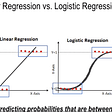## Review: PSPNet — Winner in ILSVRC 2016 (Semantic Segmentation / Scene Parsing)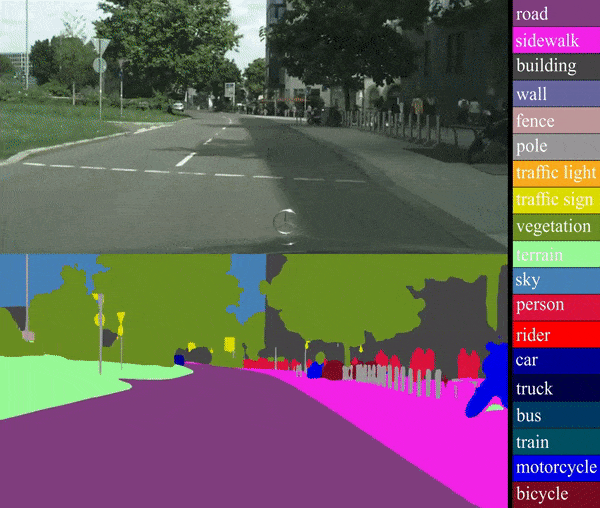## Jewelupp Instagram Paylaşımı #18251881705047091## Durham Weather Analysis## Twitter Sentiment Analysis using Natural Language Processing (NLP)## Females in Comics## Covid-19 And Viruses: Data Analysis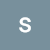## Sssiyuan

Siyuan Li, Master in Data Science at Brown University

## Batch Gradient Descent and Simple Linear Regression## Linear regression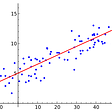## Why there are Different Cost Functions in Neural Networks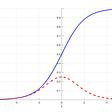## Neural Gas Simplified